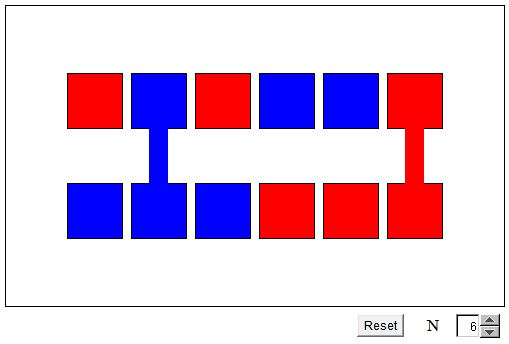# Two Colors in Two RowsWhat Is This About? A Mathematical Droodle

### If you are reading this, your browser is not set to run Java applets. Try IE11 or Safari and declare the site https://www.cut-the-knot.org as trusted in the Java setup.What if applet does not run?

ExplanationThe applet displays two rows of red and blue squares. It also joins the squares of the same color that are located in the same column. To better grasp what the applet purports to convey, you should count the number of red and blue squares in each of the two rows and the number of joined pairs of each color. You should not fail to discover that the number of red joints is always equal to the number of blue joints.

I came across this problem skimming through Barbeau et al. Problem #399 reads

Consider a rectangular array of dots with an even number of rows and an even number of columns. Color the dots, each one red or blue, subject to the condition that in each row half the dots are red and half are blue, and in each column half the dots are red and half are blue. Now, if two points are adjacent (in a row or a column) and like-colored, join them by an edge of their color. Show that the number of the blue segments is equal to the number of red segments.

A menacingly looking problem at first glance, especially if you start thinking of how to construct a required array. Luckily, as the applet suggests, one does not have to construct a big array in order to solve the problem. It is quite sufficient to consider two adjacent columns or, as in the applet, two adjacent rows. So here is a simpler problem that actually furnishes a solution to #399:

Consider two rows of dots with an even number of columns. Color the dots, each one red or blue, subject to the condition that in each row half the dots are red and half are blue. Now, if two points are adjacent in a column and like-colored, join them by an edge of their color. Show that the number of the blue segments is equal to the number of red segments.

How does the simplified problem help solve the original one? The edges are (drawn and) counted only between two adjacent rows (columns). Thus if the numbers of red and blue joints are equal between any successive pair of rows (columns), their totals are bound to be equal over the whole array. And, of course, suffice it to consider only the rows as the columns play a similar role.

Assume N = 2M is the length of a row, each of which contains M red and M blue dots. For rows r = 1, 2, introduce the following quantities:

• xr - the number of red dots in row r joined with a red dot in the other row.
• yr - the number of unmatched red dots.
• ur - the number of blue dots in row r joined with a blue dot in the other row.
• vr - the number of unmatched blue dots.

Clearly, x1 = x2, and, since yr = M - xr, we also have y1 = y2. Similarly, u1 = u2 and v1 = v2. This allows us to drop indices. Further, the number of unmatched dots of one color in a row must be the same as the number of unmatched dots of the second color in the other row. This shows that y = v. But then

x = M - y = M - v = u,

as required: the number of blue matches equals the number of red matches.

It's a nice problem that, after being stripped to its essential core, becomes almost transparent. It can also be generalized. And I believe in a surprising way at that.

(The argument is very much the same as was used with the two color solitaire game.)

### References

1. E. J. Barbeau, M. S. Klamkin, W. O. J. Moser, Five Hundred Mathematical Challenges, MAA, 1995, #399
2. R. Honsberger, Mathematical Morsels, MAA, 1978, p. 119